To prove one-one & onto (injective, surjective, bijective)

Chapter 1 Class 12 Relation and Functions
Concept wise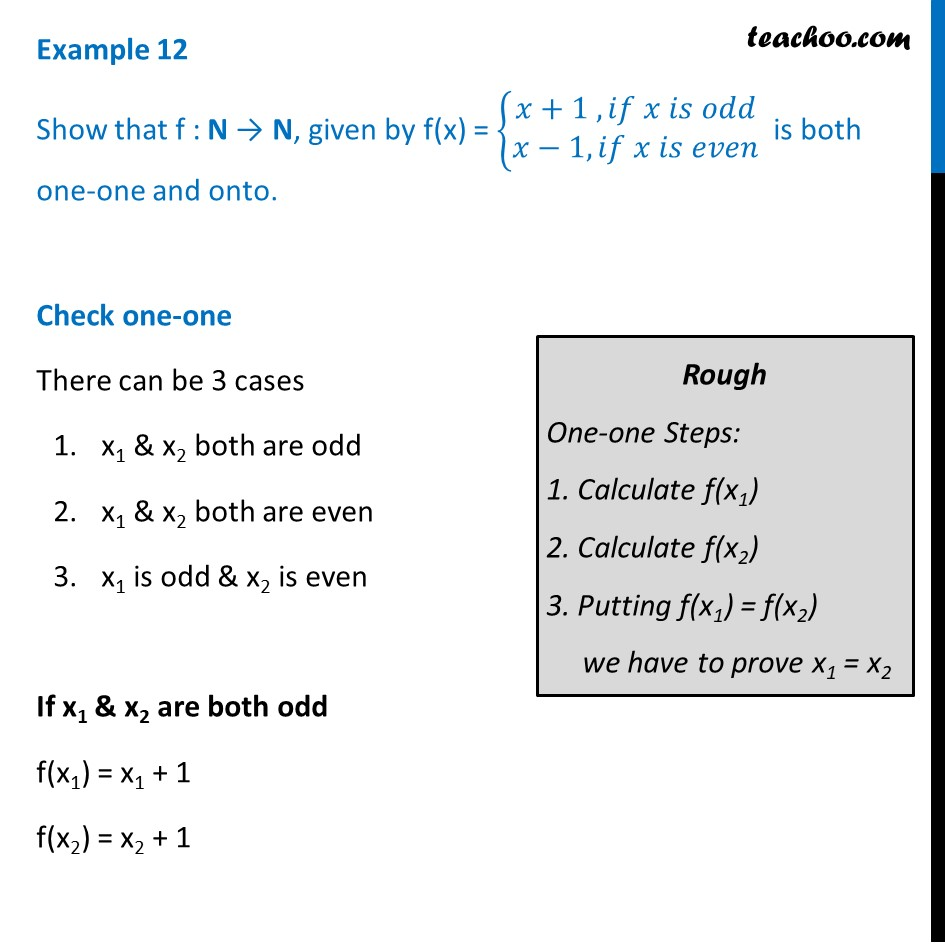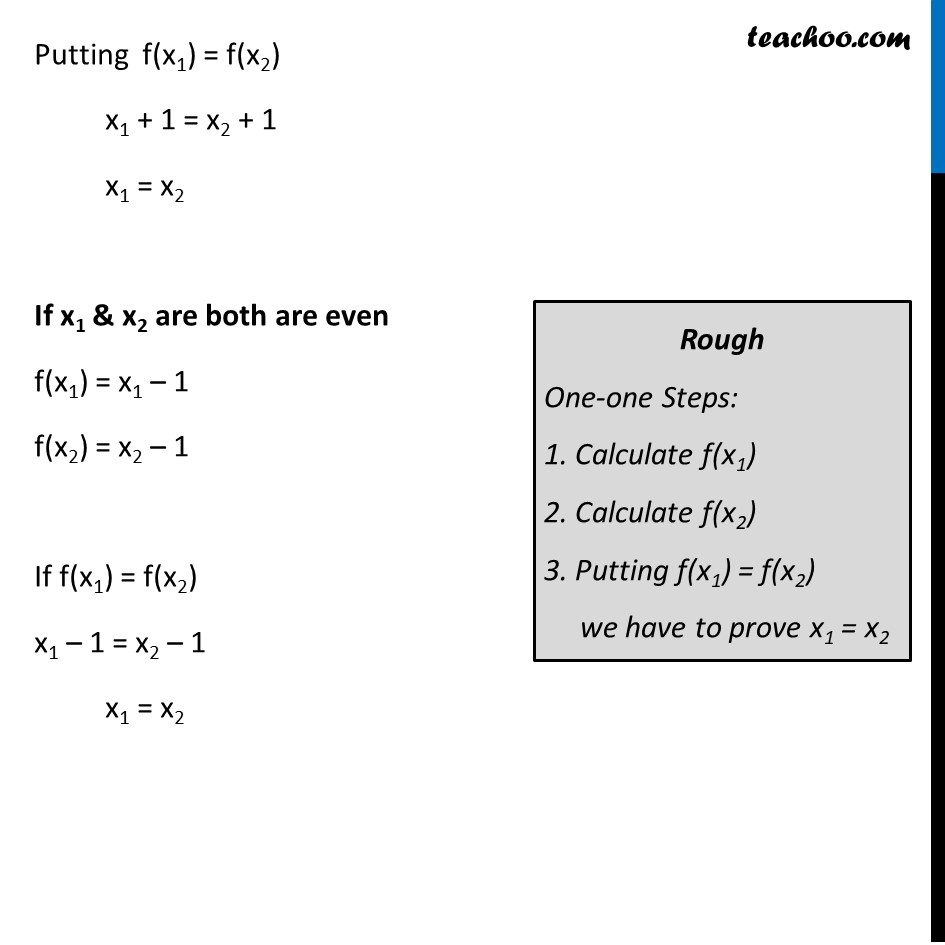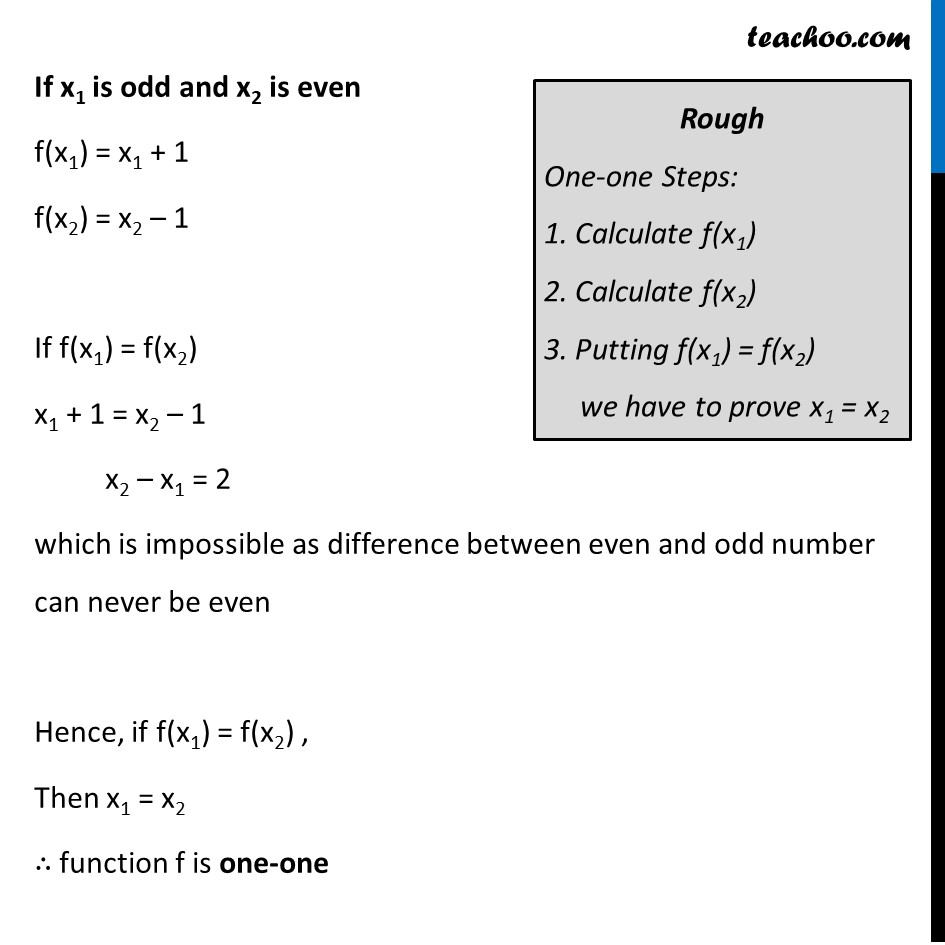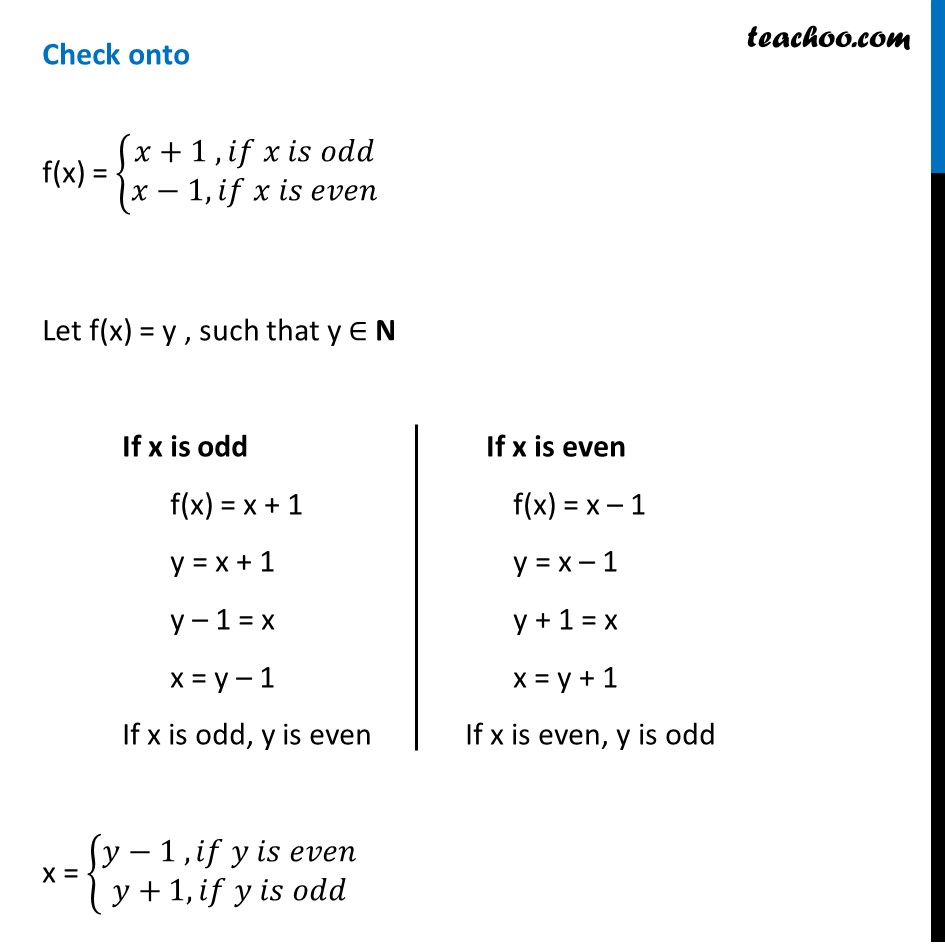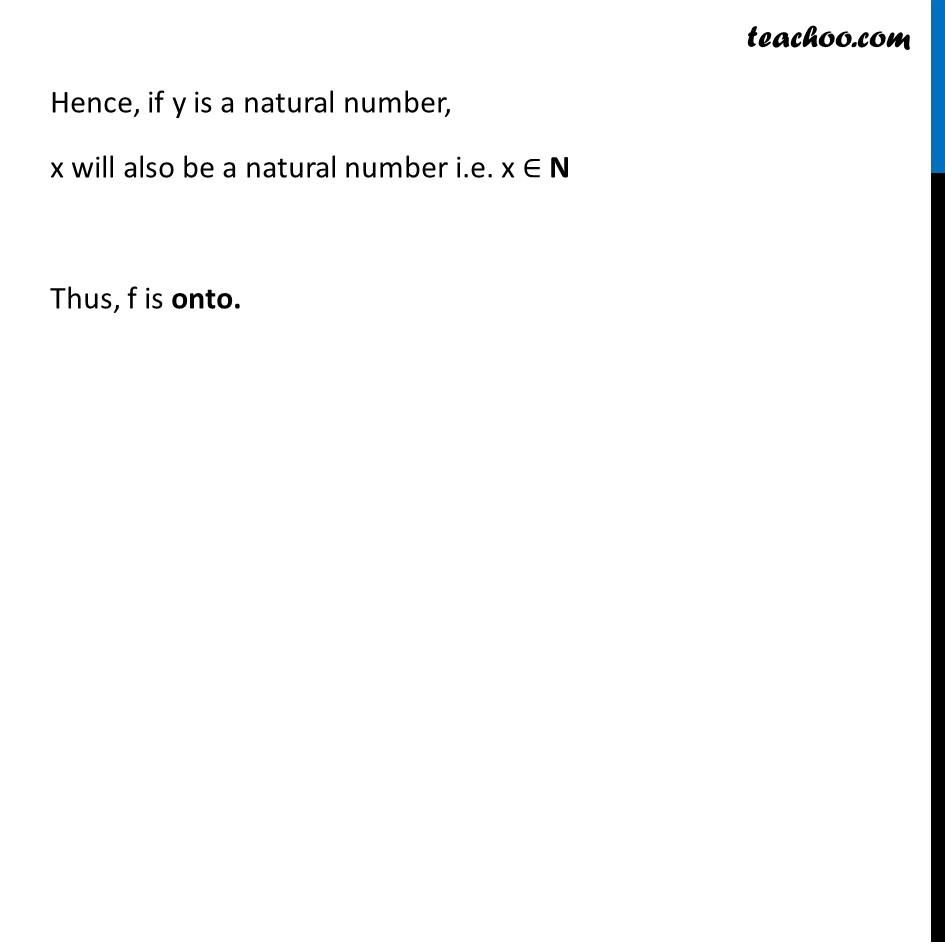Learn in your speed, with individual attention - Teachoo Maths 1-on-1 Class

### Transcript

Example 12 Show that f : N → N, given by f(x) = {█(𝑥+1 , 𝑖𝑓 𝑥 𝑖𝑠 𝑜𝑑𝑑@𝑥−1, 𝑖𝑓 𝑥 𝑖𝑠 𝑒𝑣𝑒𝑛)┤ is both one-one and onto. Check one-one There can be 3 cases x1 & x2 both are odd x1 & x2 both are even x1 is odd & x2 is even If x1 & x2 are both odd f(x1) = x1 + 1 f(x2) = x2 + 1 Putting f(x1) = f(x2) x1 + 1 = x2 + 1 x1 = x2 If x1 & x2 are both are even f(x1) = x1 – 1 f(x2) = x2 – 1 If f(x1) = f(x2) x1 – 1 = x2 – 1 x1 = x2 If x1 is odd and x2 is even f(x1) = x1 + 1 f(x2) = x2 – 1 If f(x1) = f(x2) x1 + 1 = x2 – 1 x2 – x1 = 2 which is impossible as difference between even and odd number can never be even Hence, if f(x1) = f(x2) , Then x1 = x2 ∴ function f is one-one If x is odd f(x) = x + 1 y = x + 1 y – 1 = x x = y – 1 If x is odd, y is even Check onto f(x) = {█(𝑥+1 , 𝑖𝑓 𝑥 𝑖𝑠 𝑜𝑑𝑑@𝑥−1, 𝑖𝑓 𝑥 𝑖𝑠 𝑒𝑣𝑒𝑛)┤ Let f(x) = y , such that y ∈ N x = {█(𝑦−1 , 𝑖𝑓 𝑦 𝑖𝑠 𝑒𝑣𝑒𝑛@𝑦+1, 𝑖𝑓 𝑦 𝑖𝑠 𝑜𝑑𝑑)┤ If x is even f(x) = x – 1 y = x – 1 y + 1 = x x = y + 1 If x is even, y is odd Hence, if y is a natural number, x will also be a natural number i.e. x ∈ N Thus, f is onto.